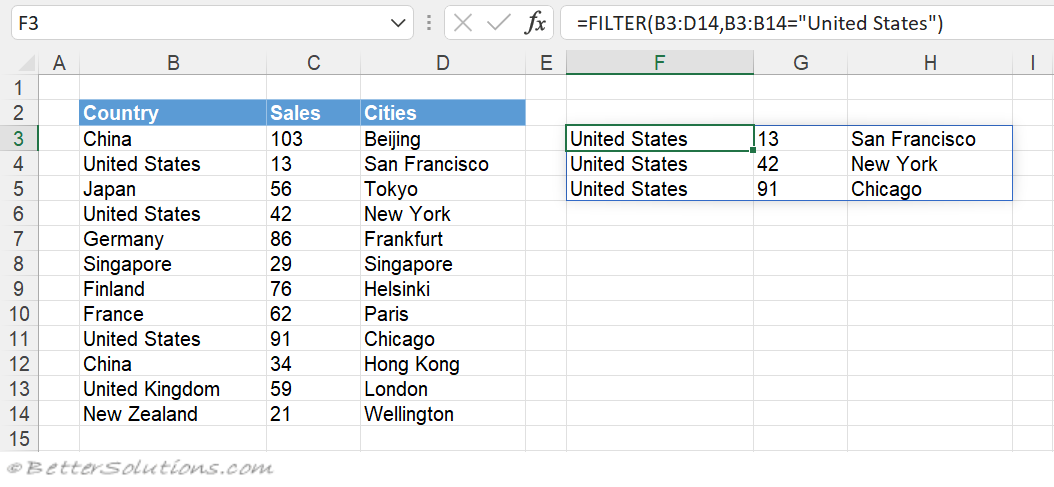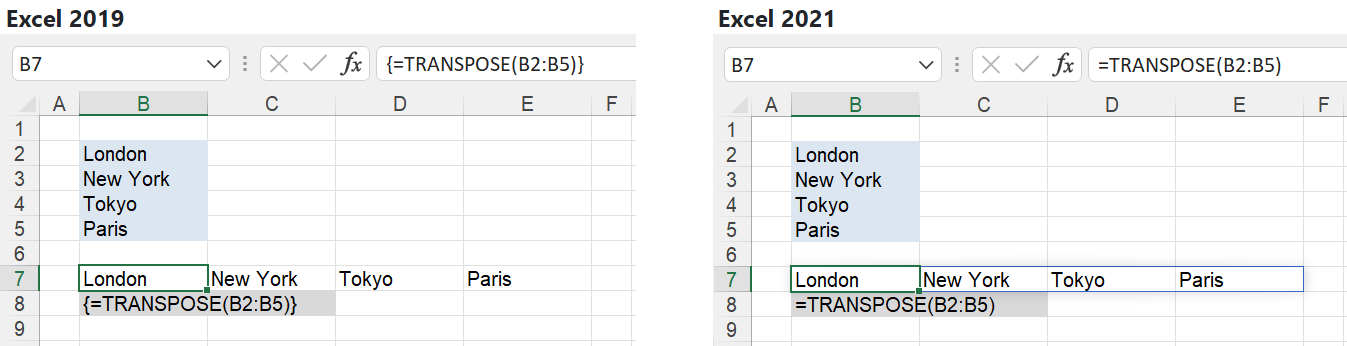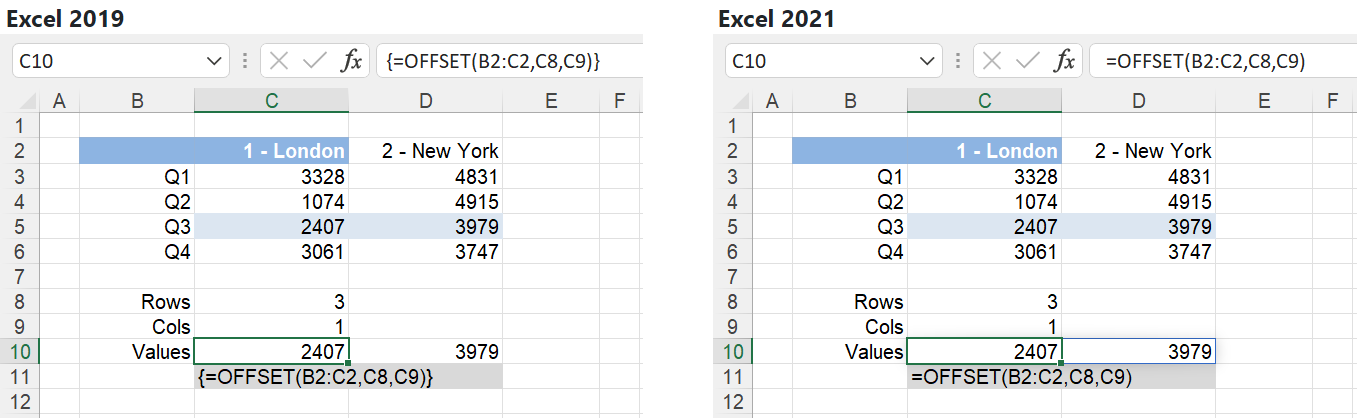# Dynamic Formulas

Introduced in Excel 2021 and also known as Dynamic Array Formulas or Spilled Array Formulas.
Formulas that return more than one value will automatically create a Dynamic Array Formula.
Formulas that return more than one value will automatically populate adjacent cells.
Formulas that return more than one value will automatically resize and update.
Formulas that return more than one value are said to spill over into adjacent cells.
Functions that return more than one value are referred to as Dynamic Array Functions.

### No More Array Formulas

These new dynamic array formulas eliminate the need for the legacy Array Formulas.
When workbooks containing dynamic formulas are opened up in previous versions of Excel they are automatically converted into array formulas.
When workbooks containing array formulas are opened up in new versions of Excel these formulas are not automatically converted into dynamic array formulas.

### Excel 2021 - New Functions

A lot of the new Functions in 2021 and Functions in 365 can return more than one value and will create dynamic array formulas.
An example of a new function added in 2021 is the FILTER function.
This function can return a dynamic array formula that contains filtered data from a range that satisfies multiple conditions.### Excel 2021 - Old Functions

In Excel 2021 any existing function from Excel 2019 that could return multiple values was also upgraded to use dynamic array formulas.
Returning more than one value in Excel 2019 was only possible when using Array Formulas
In Excel 2019 if you wanted these functions to return multiple values you had to enter then using (Ctrl + Shift + Enter).
Array formulas also required you to select the exact number of cells first, before pressing CSE.
An example of a function from Excel 2019 is the TRANSPOSE function.In Excel 2021 you type the function into one cell, press Enter and the adjacent cell(s) are automatically populated.
Another example of a function from Excel 2019 is the OFFSET function.### Microsoft Learning

Learn about array formulas with the help of this formulas tutorial template.
Use this dynamic array formulas tutorial template to complete complex tasks in Excel.
This array formulas tutorial template will teach you many efficient tips in Excel, such as calculating the cost of a loan and much more.

`link - templates.office.com/en-gb/dynamic-array-formulas-tutorial-tm67741002 link - support.office.com/en-us/article/dynamic-array-formulas-vs-legacy-cse-array-formulas-ca421f1b-fbb2-4c99-9924-df571bd4f1b4 `